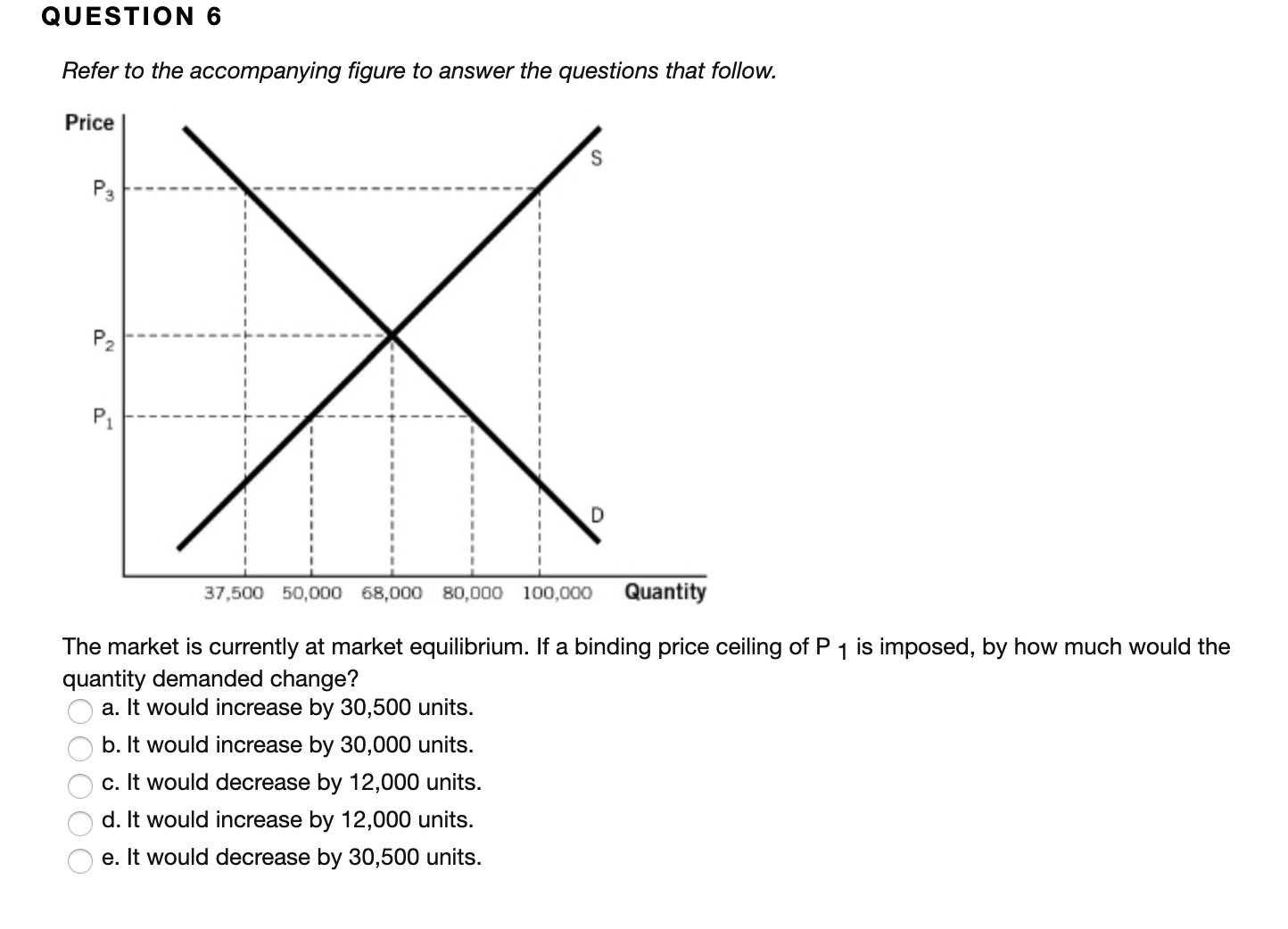# QUESTION 6Refer to the accompanying figure to answer the questions that follow.PriceP3P2P1Quantity37,500 50,000 68,000 80,000 100,000The market is currently at market equilibrium. If a binding price ceiling of P 1 is imposed, by how much would thequantity demanded change?a. It would increase by 30,500 units.b. It would increase by 30,000 units.c. It would decrease by 12,000 units.d. It would increase by 12,000 units.e. It would decrease by 30,500 units.OOOO

Question
44 viewshelp_outlineImage TranscriptioncloseQUESTION 6 Refer to the accompanying figure to answer the questions that follow. Price P3 P2 P1 Quantity 37,500 50,000 68,000 80,000 100,000 The market is currently at market equilibrium. If a binding price ceiling of P 1 is imposed, by how much would the quantity demanded change? a. It would increase by 30,500 units. b. It would increase by 30,000 units. c. It would decrease by 12,000 units. d. It would increase by 12,000 units. e. It would decrease by 30,500 units. OOOO fullscreen
check_circle

Step 1

It is given that initially the market is in equilibrium. Equilibrium exists at the point where the quantity demanded is equal to the quantity supplied. There is neither excess demand nor excess supply in the economy. In this case, equilibrium exists at price Pand quantity 68,000 units

Step 2

The quantity demanded can be determined by the intersection of the price line and the demand curve. At equilibrium, the quantity demanded is 68,000 un...

### Want to see the full answer?

See Solution

#### Want to see this answer and more?

Solutions are written by subject experts who are available 24/7. Questions are typically answered within 1 hour.*

See Solution
*Response times may vary by subject and question.
Tagged in